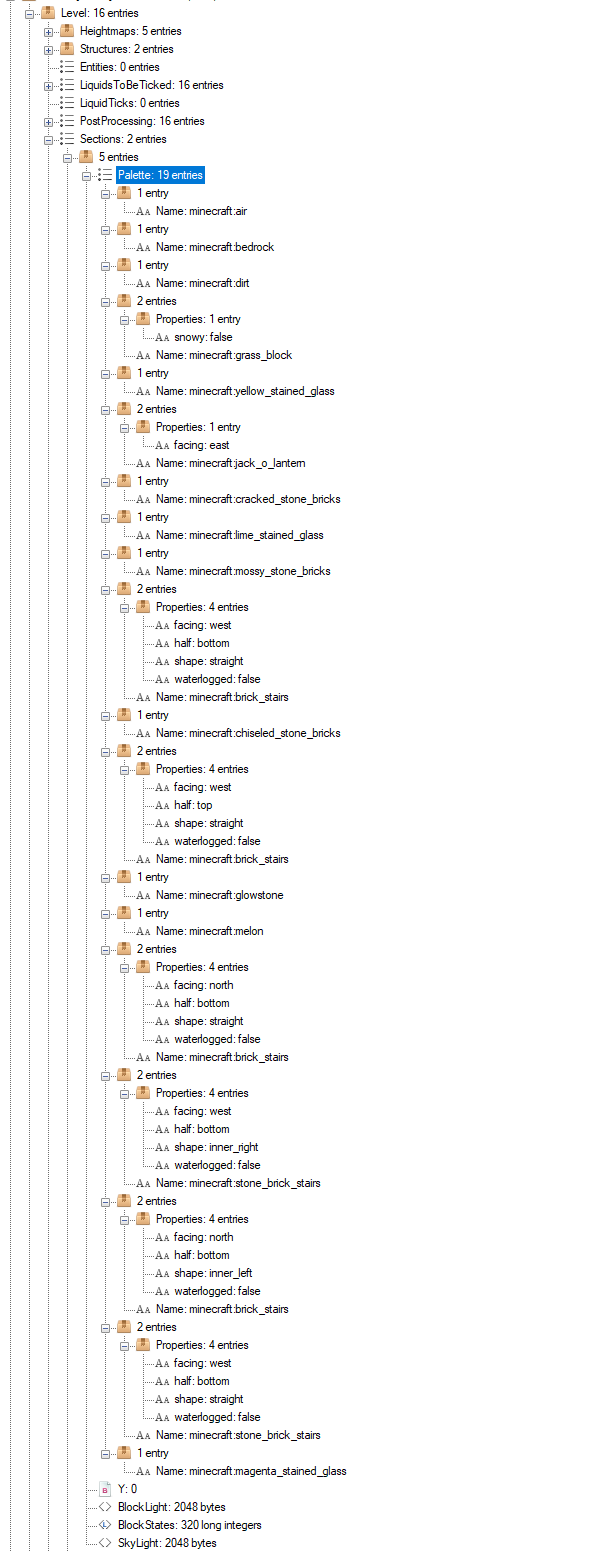# 1.13 Update¶

## Block Format¶

“Block Data” refers to information about a certain block. The two components of Block Data are its type (e.g. air, grass, acacia door, etc.) and its state. Each type has a set of properties. Each combination of all the type’s properties is a state, and has its own numerical ID.

Example:

```"minecraft:light_gray_bed": {
"properties": {
"facing": [   // Possible values for the "facing" property
"north",
"south",
"west",
"east"
],
"occupied": [ // Possible values for the "occupied" property
"true",
"false"
],
"part": [     // Possible values for the "part" property
"foot"
]
},
"states": [
{
"properties": {
"facing": "north",
"occupied": "true",
},
"id": 876
},
{
"properties": {
"facing": "north",
"occupied": "true",
"part": "foot"
},
"id": 877
},
[...]
]
}
}
```

There are 2 ways to encode Block Data:

• Numerical IDs (type base ID + state bit mask)
• Map (type’s `minecraft:...` key + map of string:string for state)

### On-Disk Block Storage¶

Each chunk section (16x16x16 blocks) has its own “Palette”. A Palette is a list of Block Data maps (see above). In NBT format, the `Palette` tag is a list of compounds.

This is an example palette for a section with 19 different block states:The `BlockStates` tag is a Long Array. Each block is represented by a Palette index, corresponding to the order of the section’s Palette tag. All indices have the same bit size, corresponding to the size required for the largest index (`ceil(log(n)/log(2))`, where `n` is the number of states in the Palette), with a minimum of 4 bits per index.

The number of longs in the tag may grow from 256 longs (16 indices per long) to as many longs as necessary to store all 4096 identically-sized indices.

### Network Representation¶

Similarly to the Disk Storage, a palette can be used to save bandwidth. The palette is a list of integers (VarInt) – the numerical IDs of each state used in the chunk.

Numerical IDs are incremental and start at 0 (`minecraft:air`). Contrary to previous Minecraft versions, these IDs are not incremental per-type, but rather per-state.

For example, if type A has 2 properties with 2 possible values each, type A has 4 possible states. If the first A state has ID 10, type B’s states will start at 14.

Encoding Example:

```"minecraft:A": {
"properties": {
"foo": [
"true",
"false"
],
"bar": [
"true",
"false",
"maybe"
]
},
"states": [
{
"properties": {
"foo": "true",
"bar": "true"
},
"id": 10
},
{
"properties": {
"foo": "true",
"bar": "false"
},
"id": 11
},
{
"properties": {
"foo": "true",
"bar": "maybe"
},
"id": 12
},
{
"properties": {
"foo": "false",
"bar": "true"
},
"id": 13
},
[...]
]
}
```

Knowing the ID of the first state of a type, it is possible to encode a state from the values of the properties.

Let’s say we want to encode `minecraft:A[foo=true,bar=maybe]` to the state’s ID (12):

1. From a pre-calculated map, we can determine the base ID corresponding to `minecraft:A` to be 10.

2. The state is encoded using a recursive algorithm:

1. A temporary value, P, is set to 0. This will be the final result once the algorithm is complete.

```P := 0
```
2. The `foo` property has 2 possible values (lenfoo = 2). The index of the value (“true”) is i = 0. P is set to the result of the following formula:

```P = i + len * P
```
3. Repeat the previous step for each property. The result for the given example will be P = 2:

```P = 2 + (0 + 2 * 0) * 3 = 2
```
4. The result is then base ID + P = 10 + 2 = 12.

A sample implementation of this algorithm can be found here (JavaScript): https://gist.github.com/momothereal/27442d5cf3b5d9ceee679b606facdfe4

Note

Decoding numerical IDs is not necessary on the server-side in 1.13.